# To draw: The graph of the function y = f ( x − 8 ) using the graph of y = f ( x ) .### Single Variable Calculus: Concepts...

4th Edition
James Stewart
Publisher: Cengage Learning
ISBN: 9781337687805### Single Variable Calculus: Concepts...

4th Edition
James Stewart
Publisher: Cengage Learning
ISBN: 9781337687805

#### Solutions

Chapter 1, Problem 10RE

(a)

To determine

## To draw:The graph of the function y=f(x−8) using the graph of y=f(x).

Expert Solution

### Explanation of Solution

Solution:

The graph of y=f(x8) can be obtained by shifting the graph y=f(x) by 8 units to the right.

Draw the graph of y=f(x8) using the given graph as shown below in Figure 1.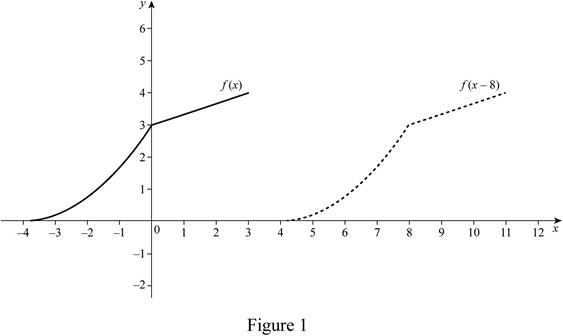From Figure 1, it is noticed that the original graph is shifted 8 units to the right.

(b)

To determine

Expert Solution

### Explanation of Solution

Solution:

The graph of y=f(x) can be obtained by reflecting the graph y=f(x) about the x-axis.

Draw the graph of y=f(x) using the given graph as shown below in Figure 2.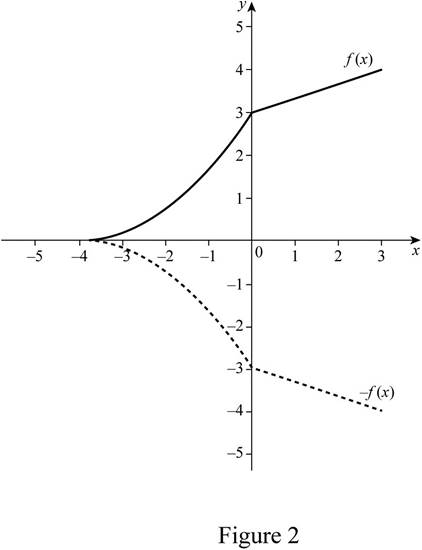From Figure 2, it is noticed that the graph y=f(x) is reflected about the x-axis.

(c)

To determine

Expert Solution

### Explanation of Solution

Solution:

The graph of y=2f(x) can be obtained by reflecting the graph y=f(x) and then shifting the graph 2 units up.

Draw the graph of y=2f(x) using the given graph as shown below in Figure 3.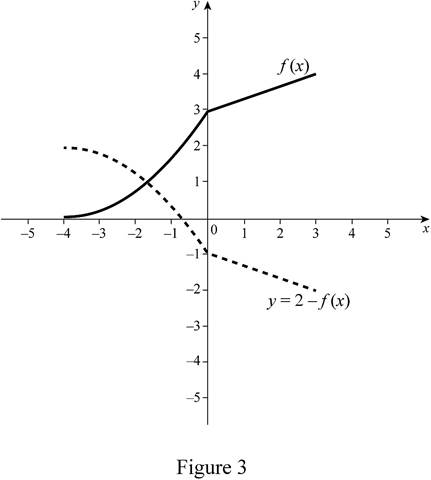Note that the reflected graph of y=f(x) is reflected and then shifted 2 units up.

(d)

To determine

Expert Solution

### Explanation of Solution

Solution:

The equation y=12f(x)1 represents that the graph of the function is shrunk vertically by a factor of 2 and then shift the graph 1 unit downward.

Draw the graph of y=12f(x)1 using the given graph as shown below in Figure 4.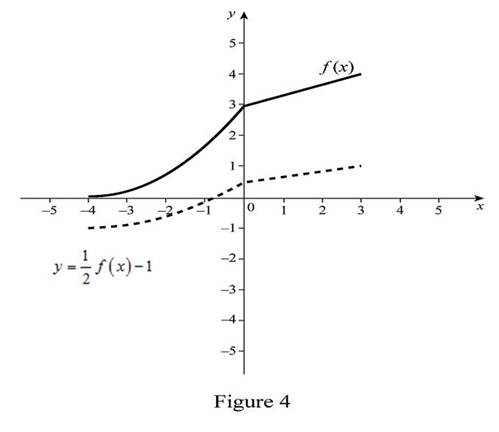From Figure 4, it is noted that the graph y=f(x) is shrunk by a factor of 2 and then shifted 1 unit down.

To determine

Expert Solution

### Explanation of Solution

Solution:

The graph of y=f1(x) can be obtained by reflecting the graph y=f(x) about the line y=x.

Draw the graph of y=f1(x) using the given graph as shown below in Figure 5.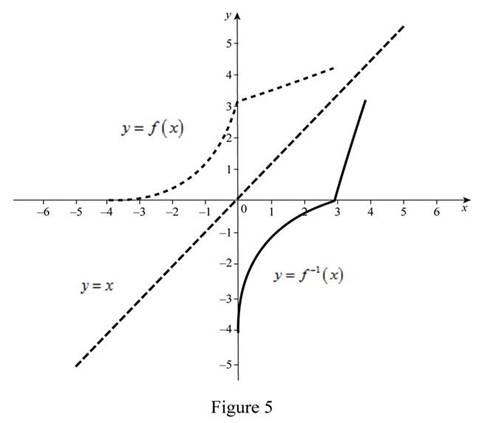From Figure 5, it is noted that the y=f(x) graph is reflected about the line y = x.

(f)

To determine

Expert Solution

### Explanation of Solution

Solution:

The graph of y=f1(x)+3 can be obtained by reflecting the graph y=f(x) about the line y=x and then shifted 3 units to the right.

Draw the graph of y=f1(x+3) using the given graph as shown below in Figure 6.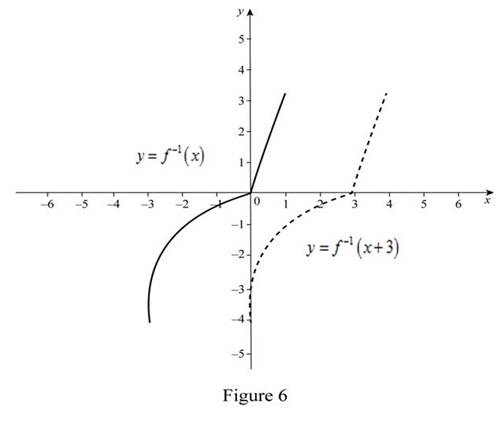From Figure 6, it is noticed that the graph of y=f(x) is reflected and then shifted three units to the right.

### Have a homework question?

Subscribe to bartleby learn! Ask subject matter experts 30 homework questions each month. Plus, you’ll have access to millions of step-by-step textbook answers!